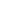Original research

# Comparison of accuracy rate in prediction of cardiovascular disease using random forest with logistic regression

## Abstract

Aim: Comparison of accuracy rate in prediction of cardiovascular disease using Novel Random Forest with Logistic Regression. Materials and Methods: The Novel Random forest (N=20) and Novel Logistic Regression Algorithm (N=20) these two algorithms are calculated by using 2 Groups and taken 20 samples for both algorithm and accuracy in this work.The sample size is determined using the G power Calculator and it’s found to be 10. Results: The Random Forest exhibited 89.06% accuracy whilst a Logistic Regression has shown 92.18%. accuracy. Statistical significance difference between Random forest algorithm and Novel Logistic Regression Algorithm was found to be p=0.001 (2 tailed) (p<0.5). Conclusion: Prediction of cardiovascular disease using Logistic Regression is significantly better than the Random Forest.

## Imprint

Talluri Vishnuvardhan, A.Rama. Comparison of Accuracy Rate in Prediction of Cardiovascular Disease using Random Forest with Logistic Regression. Cardiometry; Issue 25; December 2022; p.1526-1531; DOI: 10.18137/cardiometry.2022.25.15261531; Available from: https://www.cardiometry.net/issues/no25-december-2022/rate-prediction-cardiovascular-disease

## Keywords

Prediction of cardiovascular disease,   Novel Random forest,   Novel Logistic Regression,   Smoking,   Endocytosis,   HyperglycemiaCurrent issueCardiometry's libraryFounders of Cardiometry